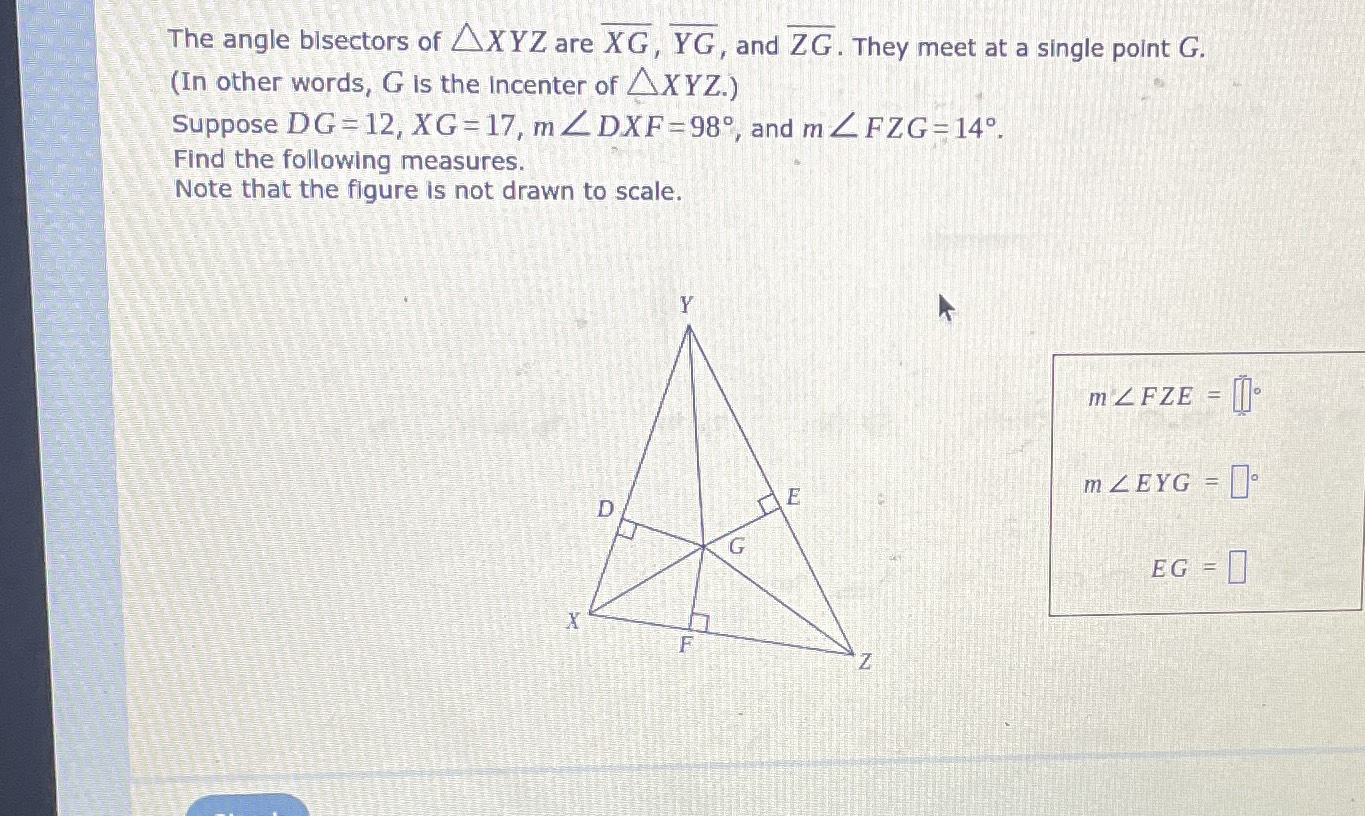### Still have math questions?

Trigonometry
QuestionThe angle bisectors of $$\triangle X Y Z$$ are $$\overline { X G } , \overline { Y G }$$ , and $$\overline { Z G }$$ . They meet at a single point $$G$$ . (In other words, $$G$$ is the incenter of $$\triangle X Y Z$$ .) Suppose $$D G = 12 , X G = 17 , m \angle D X F = 98 ^ { \circ }$$ , and $$m \angle F Z G = 14 ^ { \circ }$$ . Find the following measures. Note that the figure is not drawn to scale. $$m \angle F Z E = \square$$

$$m \angle E Y G = \square$$

$$E G = \square$$

$${M}' \angle FZE = 28$$
$$M\angle EYG= 27$$